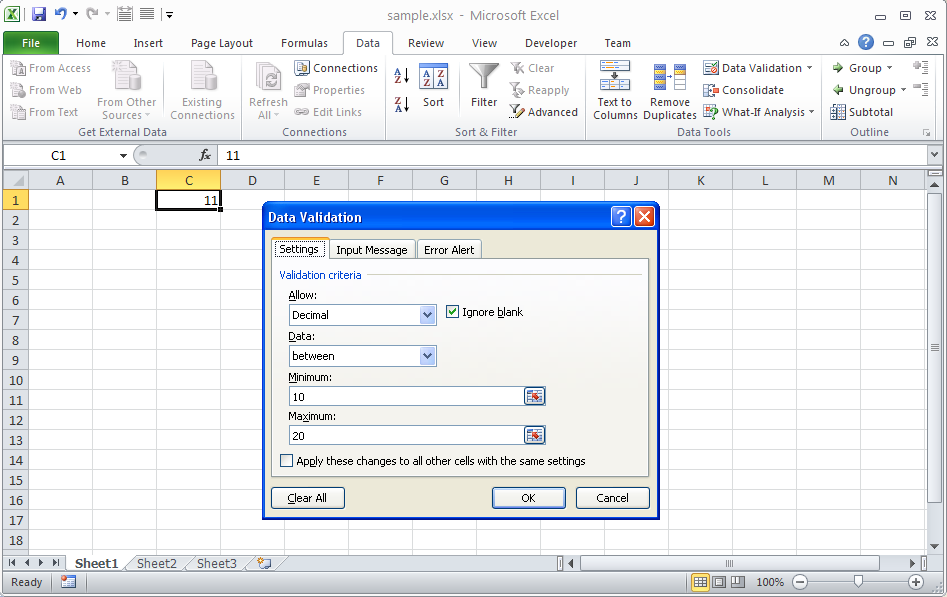# Get Validation Applied on a Cell

Contents
[ ]

The following snapshot shows the sample Microsoft Excel file used in the sample code below. Cell C1 has decimal validation applied and can only take values between 10 and 20.

A cell with validationThe sample code below gets the validation applied to C1 and reads its various properties.

Here is the console output from the sample code executed with the sample file shown in the snapshot above.

``````Reading Properties of Validation

\--------------------------------

Type: 2

Operator: 0

Formula1: =10

Formula2: =20

Ignore blank: true``````